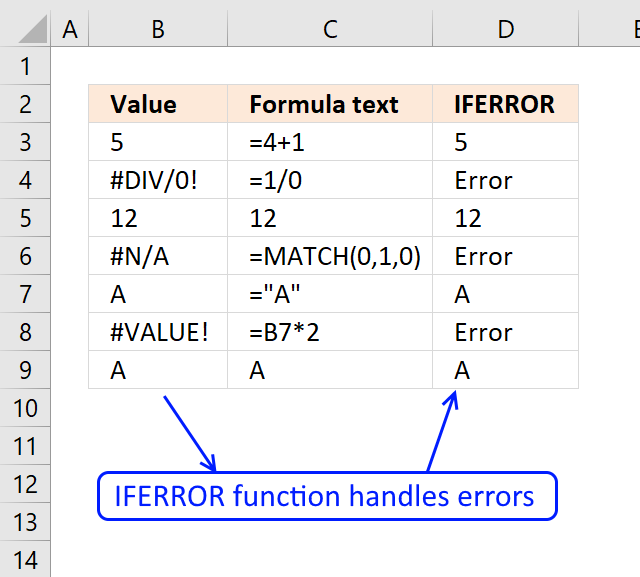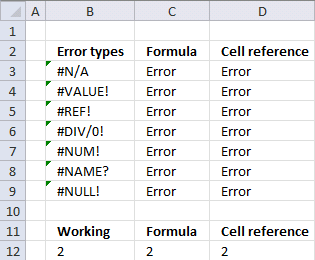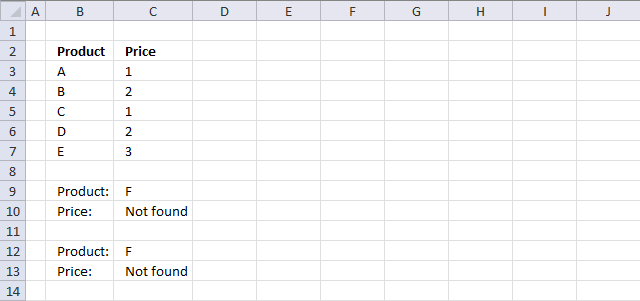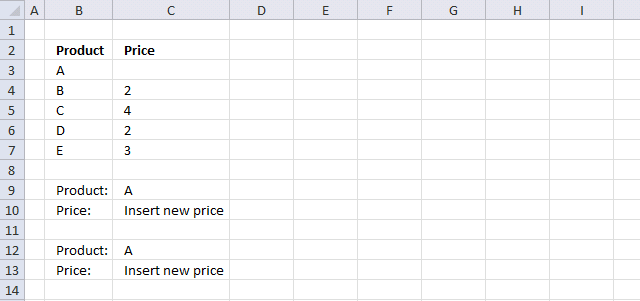Author: Oscar Cronquist Article last updated on April 17, 2018If the value argument returns an error, the value_if_error argument is used. If the value argument does NOT return an error, the IFERROR function returns the value argument.

The IFERROR function was introduced in Excel 2007. In previous Excel versions, you could check for errors with the ISERROR function.

### Excel Function Syntax

IFERROR(value, value_if_error)

### Arguments

 value A cell reference or a formula to check for errors. value_if_error the value to return if the value argument evaluates to an error. The following error types are evaluated: #N/A, #VALUE!, #REF!, #DIV/0!, #NUM!, #NAME?, or #NULL!.The number you want to remove the sign from.

### Example 1 - Trap errorsThe following formulas in column B evaluates to an error. The IFERROR functions in column C trap the errors and return value_if_error argument. In this case "Error".

The formulas in Column D contain cell references, The referenced cell (Column B) returns an error and the IFERROR functions return the value_if_error argument.

### #N/A Error

Formula in cell B2:

=MATCH(0,1,0)

Formula in cell C2:

=IFERROR(MATCH(0,1,0),"Error")

Formula in cell D2:

=IFERROR(B2,"Error")

### #VALUE Error

Formula in cell B3:

=B2*2

Formula in cell C3:

=IFERROR(B2*2,"Error")

Formula in cell D3:

=IFERROR(B3,"Error")

### #REF Error

Formula in cell B4:

=OFFSET(A1,-1,1)

Formula in cell C4:

=IFERROR(OFFSET(A1,-1,1),"Error")

Formula in cell D4:

=IFERROR(B4,"Error")

### #DIV/0 Error

Formula in cell B5:

=1/0

Formula in cell C5:

=IFERROR(1/0, "Error")

Formula in cell D5:

=IFERROR(B5, "Error")

### #NUM Error

Formula in cell B7:

=NPER(7/12,-233,40000)

Formula in cell C7:

=IFERROR(NPER(7/12,-233,40000), "Error")

Formula in cell D7:

=IFERROR(B7, "Error")

### #NAME Error

Formula in cell B8:

=Text&1

Formula in cell C8:

=IFERROR(Text&1, "Error")

Formula in cell D8:

=IFERROR(B8, "Error")

### #NULL Error

Formula in cell B9:

=F3:G4 F6:G7

Formula in cell C9:

=IFERROR(F3:G4 F6:G7, "Error")

Formula in cell D9:

=IFERROR(B9, "Error")

### IFERROR function makes formulas smaller

Example 1

In previous excel versions you could check for errors using the ISERROR function. This example shows both functions, the ISERROR function in cell C10 and IFERROR function in C12. The formula in cell C12 is much smaller.Formula in cell C10:

=IF(ISERROR(INDEX(C3:C7, MATCH(C9, B3:B7, 0))), "Not found", INDEX(C3:C7, MATCH(C9, B3:B7, 0)))

Formula in cell C13:

=IFERROR(INDEX(C3:C7, MATCH(C12, B3:B7, 0)), "Not found")

Example 2

Here the price is missing for product A. The IFERROR function simplifies the formula in cell C13.Formula in cell C10:

=IF(INDEX(C3:C7,MATCH(C9,B3:B7,0))=0,"Insert new price",INDEX(C3:C7,MATCH(C9,B3:B7,0)))

Formula in cell C13:

=IFERROR(1/(1/(INDEX(C3:C7,MATCH(C12,B3:B7,0)))),"Insert new price")

### Explaining the formula in cell C12

Step 1 - Find a value and return corresponding value

INDEX(C3:C7,MATCH(C12,B3:B7,0))

becomes

INDEX(C3:C7,MATCH("A",{"A";"B";"C";"D";"E"},0))

becomes

INDEX(C3:C7, 1)

becomes

INDEX({0;2;4;2;3}, 1)

Step 2 - Divide 1 with the returned value

=IFERROR(1/(1/(INDEX(C3:C7,MATCH(C12,B3:B7,0)))),"Insert new price")

becomes

=IFERROR(1/(1/0)),"Insert new price")

becomes

=IFERROR(1/#DIV/0),"Insert new price")

becomes

=IFERROR(#DIV/0,"Insert new price")

and returns "Insert new price" in cell C13.

### Download excel *.xlsx file

IFERROR function.xlsx